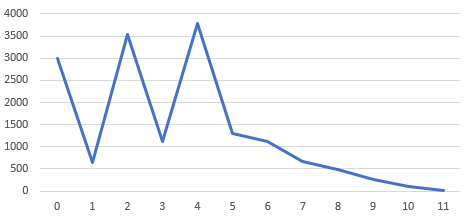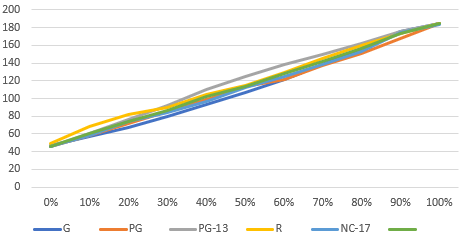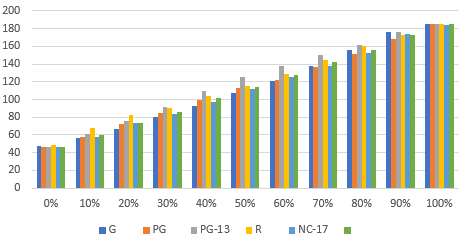## How to Emulate PERCENTILE_DISC in MySQL and Other RDBMS

In my previous article, I showed what the very useful percentile functions (also known as inverse distribution functions) can be used for. Unfortunately, these functions are not ubiquitously available in SQL dialects. As of jOOQ 3.11, they are known to work in these dialects:
DialectAs aggregate functionAs window function
Oracle 18cYesYes
PostgreSQL 11YesNo
SQL Server 2017NoYes
Oracle has the most sophisticated implementation, which supports both the ordered set aggregate function, and the window function version:
• Aggregate function: `PERCENTILE_DISC (0.5) WITHIN GROUP (ORDER BY x)`
• Window function: `PERCENTILE_DISC (0.5) WITHIN GROUP (ORDER BY x) OVER (PARTITION BY y)`

### Workarounds if the feature is unavailable

Luckily, as soon as an RDBMS supports window functions, we can easily emulate `PERCENTILE_DISC` using `PERCENT_RANK` and `FIRST_VALUE` as follows. We’re using the Sakila database in this example. Emulating window functions Let’s emulate these first, as it requires a bit less SQL transformations. This query works out of the box in Oracle:
```
SELECT DISTINCT
rating,
percentile_disc(0.5)
WITHIN GROUP (ORDER BY length)
OVER() x1,
percentile_disc(0.5)
WITHIN GROUP (ORDER BY length)
OVER (PARTITION BY rating) x2
FROM film
ORDER BY rating;

```
Yielding
```RATING  X1      X2
-------------------
G       114     107
NC-17   114     112
PG      114     113
PG-13   114     125
R       114     115
```
What we can read from this is that the median length of all films is 114 minutes, and the median lengths of films per rating range from 107 minutes to 125 minutes. I’ve used `DISTINCT` because we don’t care about visualising these values on a per-row basis in this case. This also works in SQL Server. Now, let’s assume we’re using PostgreSQL, which doesn’t support inverse distribution window functions, or MySQL, which doesn’t support inverse distribution functions at all, but both support `PERCENT_RANK` and `FIRST_VALUE`. Here’s the complete query:
```
SELECT DISTINCT
rating,
first_value(length) OVER (
ORDER BY CASE WHEN p1 <= 0.5 THEN p1 END DESC NULLS LAST) x1,
first_value(length) OVER (
PARTITION BY rating
ORDER BY CASE WHEN p2 <= 0.5 THEN p2 END DESC NULLS LAST) x2
FROM (
SELECT
rating,
length,
percent_rank() OVER (ORDER BY length) p1,
percent_rank() OVER (PARTITION BY rating ORDER BY length) p2
FROM film
) t
ORDER BY rating;

```
So, we’re doing this in two steps (visual example further down):
1. `PERCENT_RANK`: In a derived table, we’re calculating the `PERCENT_RANK` value, which attributes a rank to each row ordered by length, going from 0 to 1. This makes sense. When looking for the median value, we’re really looking for the value whose `PERCENT_RANK` is 0.5 or less. When looking for the 90% percentile, we’re looking for the value whose `PERCENT_RANK` is 0.9 or less
2. `FIRST_VALUE`: Once we’ve found the `PERCENT_RANK`, we’re not quite done yet. We need to find the last row whose `PERCENT_RANK` is less or equal to the percentile we’re interested in. I could have used `LAST_VALUE`, but then I would have needed to resort to using the quite verbose range clause of window functions. Instead, I when ordering the rows by `PERCENT_RANK` (p1 or p2), I translated all ranks higher than the percentile I’m looking for into `NULL` using a `CASE` expression, and then I made sure using `NULLS LAST` that the percentile I’m looking for will be the first row in the `FIRST_VALUE` function’s window specification. Easy!
To visualise this, let’s run these queries, which also project the p1 and p2 values respectively:
```
SELECT
length,
CASE WHEN p1 <= 0.5 THEN p1 END::numeric(3,2) p1,
first_value(length) OVER (
ORDER BY CASE WHEN p1 <= 0.5 THEN p1 END DESC NULLS LAST) x1
FROM (
SELECT
length,
percent_rank() OVER (ORDER BY length) p1
FROM film
) t
ORDER BY length;

```
The result is
```length |p1   |x1  |
-------|-----|----|
46     |0.00 |114 |
46     |0.00 |114 |
46     |0.00 |114 |
46     |0.00 |114 |
46     |0.00 |114 |
47     |0.01 |114 |
...
113    |0.49 |114 |
114    |0.49 |114 |
114    |0.49 |114 |
114    |0.49 |114 |
114    |0.49 |114 |
114    |0.49 |114 |
114    |0.49 |114 |
114    |0.49 |114 |
114    |0.49 |114 |
114    |0.49 |114 |
114    |0.49 |114 | <-- Last row whose PERCENT_RANK is <= 0.5
115    |     |114 |
115    |     |114 |
115    |     |114 |
115    |     |114 |
115    |     |114 |
115    |     |114 |
...
185    |     |114 |
185    |     |114 |
185    |     |114 |
```
So the `FIRST_VALUE` function just searches for that first row (descendingly, i.e. bottom up) whose p1 value is non-null. The same for p2:
```
SELECT
length,
rating,
CASE WHEN p2 <= 0.5 THEN p2 END::numeric(3,2) p2,
first_value(length) OVER (
PARTITION BY rating
ORDER BY CASE WHEN p2 <= 0.5 THEN p2 END DESC NULLS LAST) x2
FROM (
SELECT
rating,
length,
percent_rank() OVER (PARTITION BY rating ORDER BY length) p2
FROM film
) t
ORDER BY rating, length;

```
Yielding:
```length |rating |p2   |x2  |
-------|-------|-----|----|
47     |G      |0.00 |107 |
47     |G      |0.00 |107 |
48     |G      |0.01 |107 |
48     |G      |0.01 |107 |
...
105    |G      |0.47 |107 |
106    |G      |0.49 |107 |
107    |G      |0.49 |107 |
107    |G      |0.49 |107 | <-- Last row in G partition whose
108    |G      |     |107 |     PERCENT_RANK is <= 0.5
108    |G      |     |107 |
109    |G      |     |107 |
...
185    |G      |     |107 |
185    |G      |     |107 |
46     |PG     |0.00 |113 |
47     |PG     |0.01 |113 |
47     |PG     |0.01 |113 |
...
111    |PG     |0.49 |113 |
113    |PG     |0.49 |113 |
113    |PG     |0.49 |113 | <-- Last row in PG partition whose
114    |PG     |     |113 |     PERCENT_RANK is <= 0.5
114    |PG     |     |113 |
...
```
Perfect! Notice if your RDBMS doesn’t support the `NULLS LAST` clause in your `ORDER BY` clause (e.g. MySQL), you might either hope that it defaults to sorting `NULLS LAST` (MySQL does), or you can emulate it as such:
```
-- This
ORDER BY x NULLS LAST

-- Is the same as this
ORDER BY
CASE WHEN x IS NULL THEN 1 ELSE 0 END,
x

```
Emulating aggregate functions If you’re using SQL Server and want aggregate function behaviour, I recommend using the window function instead and emulate aggregation using `DISTINCT`. It will probably be easier than the emulation below. Do check for performance though! When you’re using e.g. MySQL, which doesn’t have inverse distribution function support at all, then this chapter is for you. Here’s how to use the aggregate function version in Oracle:
```
-- Without GROUP BY
SELECT percentile_disc(0.5) WITHIN GROUP (ORDER BY length) x1
FROM film;

-- With GROUP BY
SELECT
rating,
percentile_disc(0.5) WITHIN GROUP (ORDER BY length) x2
FROM film
GROUP BY rating
ORDER BY rating;

```
Trivial! The result is the same as before:
```X1
---
114

RATING  X2
-----------
G       107
NC-17   112
PG      113
PG-13   125
R       115
```
Now, let’s emulate these on e.g. MySQL, using window functions.
```
-- Without GROUP BY
SELECT
MAX(x1) x1
FROM (
SELECT first_value(length) OVER (
ORDER BY CASE WHEN p1 <= 0.5 THEN p1 END DESC NULLS LAST) x1
FROM (
SELECT
length,
percent_rank() OVER (ORDER BY length) p1
FROM film
) t
) t;

```
It’s exactly the same technique as before, except we now have to turn the window function behaviour (don’t group, preserve rows, repeat aggregation value on each row) back into aggregate function behaviour (group, collapse rows) by using an aggregate function, such as `MAX()`. This is the same as what I did before with `DISTINCT`, for illustration purposes.
```
-- With GROUP BY
SELECT
rating,
MAX(x2) x2
FROM (
SELECT
rating,
first_value(length) OVER (
PARTITION BY rating
ORDER BY CASE WHEN p2 <= 0.5 THEN p2 END DESC NULLS LAST) x2
FROM (
SELECT
rating,
length,
percent_rank() OVER (
PARTITION BY rating
ORDER BY length) p2
FROM film
) t
) t
GROUP BY rating
ORDER BY rating;

```
All we’re really doing (again) is translate the `GROUP BY` expression to a `PARTITION BY` expression in the window function, and then redo the previous exercise.

### Conclusion

Window functions are extremely powerful. They can be used and combined to calculate a variety of other aggregations. With the above approach, we can calculate the `PERCENTILE_DISC` inverse distribution function, which is not readily available in most RDBMS using a more verbose but equally powerful approach that uses `PERCENT_RANK` and `FIRST_VALUE` in all RDBMS that support window functions. A similar exercise could be made with `PERCENTILE_CONT` with a slightly more tricky approach to finding that `FIRST_VALUE`, which I’ll leave as an exercise to the reader. A future jOOQ version might emulate this for you, automatically. Liked this article? You may also like 10 SQL Tricks That You Didn’t Think Were Possible.

## Calculate Percentiles to Learn About Data Set Skew in SQL

B-Tree indexes are perfect when your data is uniformly distributed. They are not really useful, when you have skewed data. I’ll explain later why this is the case, but let’s first learn how to detect “skew”

### What is skew?

Skew is a term from statistics when a normal distribution is not symmetric. The example given on Wikipedia shows a distribution like this:In RDBMS, we sometimes use the term skew colloquially to mean the same thing as non-uniform distribution, i.e. a normal distribution would also be skewed. We simply mean that some values appear more often than others. Thus, I will put the term “skew” in double quotes in this article. While your RDBMS’s statistics contain this information once they are calculated, we can also detect such “skew” manually in ad-hoc queries using percentiles, which are defined in the SQL standard and supported in a variety of databases, as ordinary aggregate functions, including:
• Oracle
• PostgreSQL
• SQL Server (regrettably, only as window functions)
Uniform distribution Let’s look at the FILM_ID values in the Sakila database:
```
SELECT
percentile_disc(0.0) WITHIN GROUP (ORDER BY film_id) AS "0%",
percentile_disc(0.1) WITHIN GROUP (ORDER BY film_id) AS "10%",
percentile_disc(0.2) WITHIN GROUP (ORDER BY film_id) AS "20%",
percentile_disc(0.3) WITHIN GROUP (ORDER BY film_id) AS "30%",
percentile_disc(0.4) WITHIN GROUP (ORDER BY film_id) AS "40%",
percentile_disc(0.5) WITHIN GROUP (ORDER BY film_id) AS "50%",
percentile_disc(0.6) WITHIN GROUP (ORDER BY film_id) AS "60%",
percentile_disc(0.7) WITHIN GROUP (ORDER BY film_id) AS "70%",
percentile_disc(0.8) WITHIN GROUP (ORDER BY film_id) AS "80%",
percentile_disc(0.9) WITHIN GROUP (ORDER BY film_id) AS "90%",
percentile_disc(1.0) WITHIN GROUP (ORDER BY film_id) AS "100%"
FROM film;

```
What are we calculating here? We’re trying to find 11 different values for which we can say that:
• 0% of the film_ids are lower than the “0%” value
• 10% of the film_ids are lower than the “10%” value
Or in other words:
• 0% is the MIN(film_id) value
• 50% is the MEDIAN(film_id) value
• 100% is the MAX(film_id) value
The result shows an unsurprisingly uniform distribution:
```0% |10% |20% |30% |40% |50% |60% |70% |80% |90% |100% |
---|----|----|----|----|----|----|----|----|----|-----|
1  |100 |200 |300 |400 |500 |600 |700 |800 |900 |1000 |
```
We can plot this in Microsoft Excel or some other tool to get this nice curve:This is not surprising, as the IDs are just consecutive values, which is a desired property of surrogate keys. “Skewed” distribution It’s a different story when we look at the distribution of amounts in the payment table:
```
SELECT
percentile_disc(0.0) WITHIN GROUP (ORDER BY amount) AS "0%",
percentile_disc(0.1) WITHIN GROUP (ORDER BY amount) AS "10%",
percentile_disc(0.2) WITHIN GROUP (ORDER BY amount) AS "20%",
percentile_disc(0.3) WITHIN GROUP (ORDER BY amount) AS "30%",
percentile_disc(0.4) WITHIN GROUP (ORDER BY amount) AS "40%",
percentile_disc(0.5) WITHIN GROUP (ORDER BY amount) AS "50%",
percentile_disc(0.6) WITHIN GROUP (ORDER BY amount) AS "60%",
percentile_disc(0.7) WITHIN GROUP (ORDER BY amount) AS "70%",
percentile_disc(0.8) WITHIN GROUP (ORDER BY amount) AS "80%",
percentile_disc(0.9) WITHIN GROUP (ORDER BY amount) AS "90%",
percentile_disc(1.0) WITHIN GROUP (ORDER BY amount) AS "100%"
FROM payment;

```
We’re now getting:
```0%   |10%  |20%  |30%  |40%  |50%  |60%  |70%  |80%  |90%  |100%
-----|-----|-----|-----|-----|-----|-----|-----|-----|-----|-----
0.00 |0.99 |1.99 |2.99 |2.99 |3.99 |4.99 |4.99 |5.99 |6.99 |11.99
```
This looks … “skewed”, although clearly the bias is mainly caused by the fact that this data is generated. When we plot the above, we’re getting:The slope is less steep at the beginning of this curve, which essentially means that more values exist at the lower end of the range than at the upper end. We can validate this with another query:
```
SELECT amount, count(*)
FROM (
SELECT trunc(amount) AS amount
FROM payment
) t
GROUP BY amount
ORDER BY amount;

```
… which yields:
```amount |count |
-------|------|
0      |3003  |
1      |641   |
2      |3542  |
3      |1117  |
4      |3789  |
5      |1306  |
6      |1119  |
7      |675   |
8      |486   |
9      |257   |
10     |104   |
11     |10    |
```
Plotted:When plotting this, we can see that there are more amounts in the lower half of the range than in the upper half, which leads to percentiles growing slower. Correlations This technique can also be applied to detect correlations in data. We can, for instance, try to find the percentiles of the length of films, and group data sets by rating. I’m using a GROUPING SETS function here, the ROLLUP() function, to calculate the grand total as well. Just check out the query and its results, and you’ll see:
```
SELECT
rating,
count(*),
percentile_disc(0.0) WITHIN GROUP (ORDER BY length) AS "0%",
percentile_disc(0.1) WITHIN GROUP (ORDER BY length) AS "10%",
percentile_disc(0.2) WITHIN GROUP (ORDER BY length) AS "20%",
percentile_disc(0.3) WITHIN GROUP (ORDER BY length) AS "30%",
percentile_disc(0.4) WITHIN GROUP (ORDER BY length) AS "40%",
percentile_disc(0.5) WITHIN GROUP (ORDER BY length) AS "50%",
percentile_disc(0.6) WITHIN GROUP (ORDER BY length) AS "60%",
percentile_disc(0.7) WITHIN GROUP (ORDER BY length) AS "70%",
percentile_disc(0.8) WITHIN GROUP (ORDER BY length) AS "80%",
percentile_disc(0.9) WITHIN GROUP (ORDER BY length) AS "90%",
percentile_disc(1.0) WITHIN GROUP (ORDER BY length) AS "100%"
FROM film
GROUP BY ROLLUP(rating);

```
This yields:
```rating |count |0% |10% |20% |30% |40% |50% |60% |70% |80% |90% |100% |
-------|------|---|----|----|----|----|----|----|----|----|----|-----|
G      |178   |47 |57  |67  |80  |93  |107 |121 |138 |156 |176 |185  |
PG     |194   |46 |58  |72  |85  |99  |113 |122 |137 |151 |168 |185  |
PG-13  |223   |46 |61  |76  |92  |110 |125 |138 |150 |162 |176 |185  |
R      |195   |49 |68  |82  |90  |104 |115 |129 |145 |160 |173 |185  |
NC-17  |210   |46 |58  |74  |84  |97  |112 |125 |138 |153 |174 |184  |
|1000  |46 |60  |74  |86  |102 |114 |128 |142 |156 |173 |185  |
```
So, the `GROUP BY` clause produced one row per rating, and an additional grand total column at the bottom. For illustration purposes, I’ve added the `COUNT(*)` column, to show how many films are in each group. The 5 first rows sum up to 1000, which is again the grand total at the bottom. Let’s plot the percentiles now as line and bar charts:We can “see” that there is no strong correlation between the two data points. Both data sets are close to uniformly distributed, quite independently of the rating, with the exception of PG-13, which is just slightly skewed towards longer film lengths. Again, this isn’t terribly interesting as the data set was generated, probably using some randomness to avoid perfectly uniform distribution. In real world scenarios, the above data would have been more “skewed”.

### How does this help with performance?

A balanced tree index is very useful when data is quite uniformly distributed, because in that case, it can help access data points or ranges of data in O(log(N)) time. This is quite a useful property for queries that look for film_id values, e.g.
```
SELECT *
FROM film
WHERE film_id = 1

```
When accessing “skewed” data, some values are more equal than others. This means that for example if we’re looking for amounts in the payment table, these two queries are not the same:
```
-- A lot of rows returned (3644)
SELECT * FROM payment WHERE amount BETWEEN 0 AND 2;

-- Few rows returned (361)
SELECT * FROM payment WHERE amount BETWEEN 9 AND 11;

```
An index on the amount column could have been useful for the second query, but maybe not for the first one. There are several things we can do to make sure optimal index usage is being applied for all sorts of queries. In case of uniformly distributed data, we usually don’t have to do anything as SQL developers. In case of “skewed” data sets, it may be worth thinking about:
• Using histogram statistics
• Hinting the optimiser (in Oracle or SQL Server)
• Avoiding bind variables (only in extreme cases)

### Conclusion

Not all data sets are equal. They are often “skewed”. By “skewed”, in SQL, we don’t mean the statistical meaning of a normal distribution being skewed asymmetrically. We mean that a distribution is not uniform, so even a normal distribution is “skewed”. When it is, then some values appear way more often than others. Some examples are: Uniform distribution
• Surrogate keys generated from sequences (consecutive)
• Surrogate keys generated from UUIDs (random)
• Foreign keys on one-to-one relationships
Slight “skew”
Possibly significant “skew” This really depends on the actual data set, but do expect significant “skew” in these data types
• Foreign keys on to-many relationships (e.g. some customers have more assets than others)
• Numeric values (e.g. amount)
• Codes and other discrete values (e.g. film rating, payment settlement codes, etc.)
This article has shown how we can use simple SQL aggregate functions, including the percentiles, to calculate and visualise such “skew”.